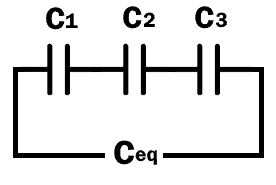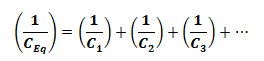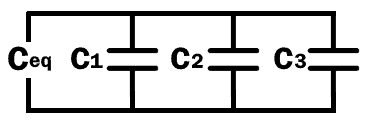# Resistance, Capacitance & Inductance in Series-Parallel – Equation & Formulas

## Resistor, Capacitor and Inductor in Series & Parallel – Formulas & Equations

The following basic and useful equation and formulas can be used to design, measure, simplify and analyze the electric circuits for different components and electrical elements such as resistors, capacitors and inductors in series and parallel combination.### Resistance in Series & Parallel Equations

Resistance:

The total equivalent resistance of resistors connected in series or parallel configuration is given the following formulas:

#### Resistance In Series:When two or more than two resistors are connected in series as shown in figure their equivalent resistance is calculated by:

REq = R1 + R2 + R3 +… Rn

#### Resistance In Parallel:when the resistors are in parallel configuration the equivalent resistance becomes:Where

• REq is the equivalent resistance of all resistors (R1, R2, R3…Rn)

Related Posts:

#### Delta Δ to Wye Y (Pi to Tee) Conversion:

The delta (Δ) interconnection is also referred to as Pi interconnection & the wye (Y) interconnection is also referred to as Tee (T) interconnection.From Delta (Δ) to Wye (Y) Interconnection:From Wye (Y) to Delta (Δ) InterconnectionFor more details and solved examples, check the Star to Delta and Delta to Star transformation.

### Capacitance in Series & Parallel Equations

Capacitance:

Total capacitance of the capacitor connected in parallel & series configuration are given below:

#### Capacitance In Series:When the capacitors are connected in series configuration the equivalent capacitance becomes:#### Capacitance In Parallel:The capacitance sums up together when they are connected together in a parallel configuration

CEq = C1 + C2 + C3 +… Cn

Where

• CEq is the equivalent Capacitance of all capacitors (C1, C2, C3…Cn)

Related Posts:

### Inductance in Series & Parallel Equations

Inductance:

The calculation of total Inductance of inductors inside a circuit resembles resistors.

#### Inductance In Series:When the inductors are in series as shown in the figure, their inductance adds up together.

LEq = L1 + L2 + L3 +… Ln

#### Inductance In Parallel:In parallel combination, the equivalent Inductance of the inductors is given byWhere

• LEq is the equivalent Inductance of all inductors (L1, L2, L3…Ln)

Related Formulas and Equations Posts: